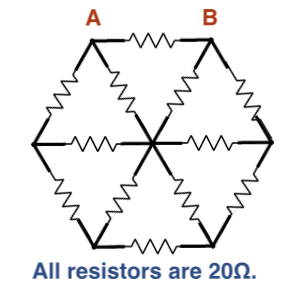# Hexagonal OhmTwelve identical wires are connected in the plane as shown in the figure above. The six outer wires make a regular hexagon and the remaining six join the vertices of this hexagon with the hexagon's center. Each wire has a resistance of $20 \Omega$. Calculate effective resistance between $A$ and $B$.

×